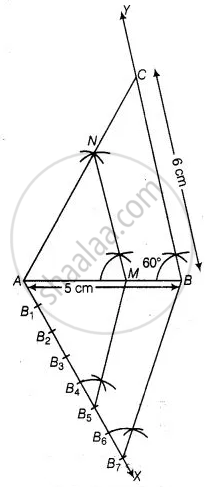# Draw a triangle ABC in which AB = 5 cm, BC = 6 cm and ∠ABC = 60°. Construct a triangle similar to ∆ABC with scale factor 57. Justify the construction. - Mathematics

Diagram
Sum

Draw a triangle ABC in which AB = 5 cm, BC = 6 cm and ∠ABC = 60°. Construct a triangle similar to ∆ABC with scale factor 5/7. Justify the construction.

#### Solution

Steps of construction:

1. Draw a line segment AB = 5 cm.

2. From point B, draw ∠ABY = 60° on which take BC = 6 cm.

3. Join AC, ∆ABC is the required triangle.

4. From A, draw any ray AX downwards making an acute angle.

5. Mark 7 points B1, B2, B3, B4, B5, B6 and B7 on AX, such that AB1 = B1B2 = B2B3 = B3B4 = B4B5 = B5B6= B6B7.

6. Join B7B and from B5 draw B5M ॥ B7B intersecting AB at M.

7. From point M draw MN ॥ BC intersecting AC at N. Then, ∆AMN is the required triangle whose sides are equal to 5/7 of the corresponding sides of the ∆ABC.Justification:

Here, B5M ॥ B7B  .....(By construction)

∴ (AM)/(MB) = 5/2

Now, (AB)/(AM) = (AM + MB)/(AM)

= 1 + (MB)/(AM) = 1 + 2/5 = 7/5

Also, MN || BC

∴ ∆AMN ∼ ∆ABC

Therefore, (AM)/(AB) = (AN)/(AC) = (NM)/(BC) = 5/7

Concept: Division of a Line Segment
Is there an error in this question or solution?

#### APPEARS IN

NCERT Mathematics Exemplar Class 10
Chapter 10 Construction
Exercise 10.4 | Q 5 | Page 117
Share